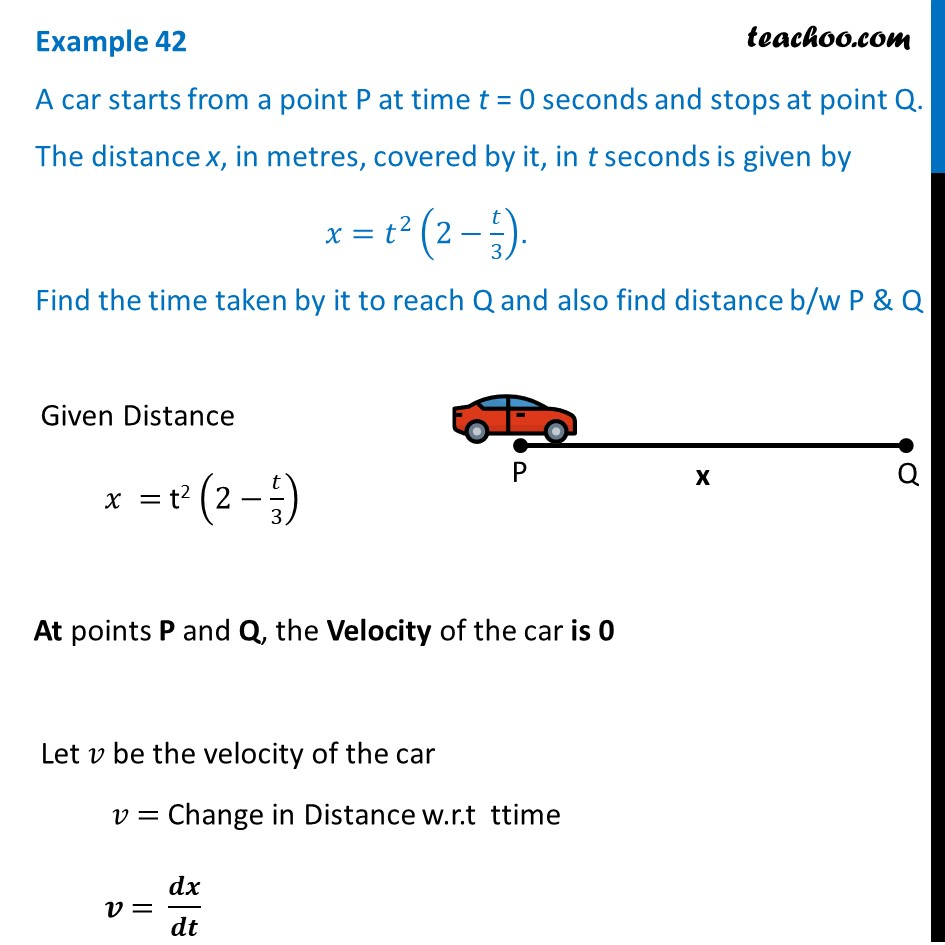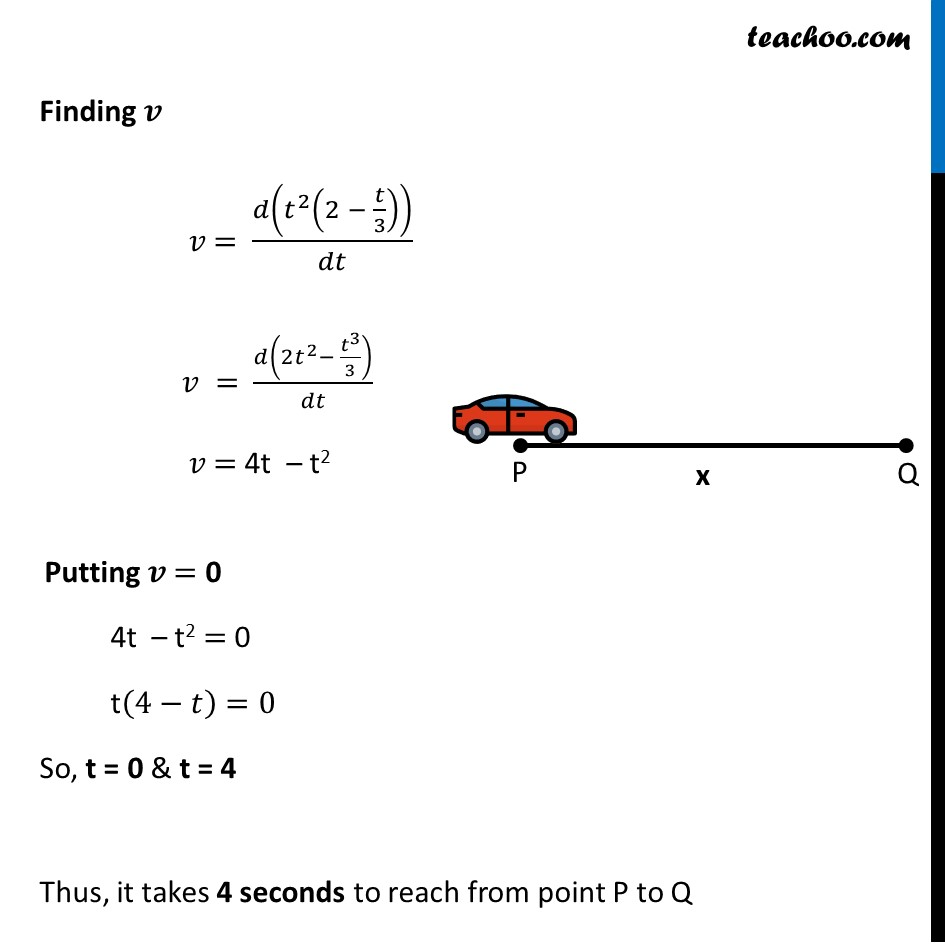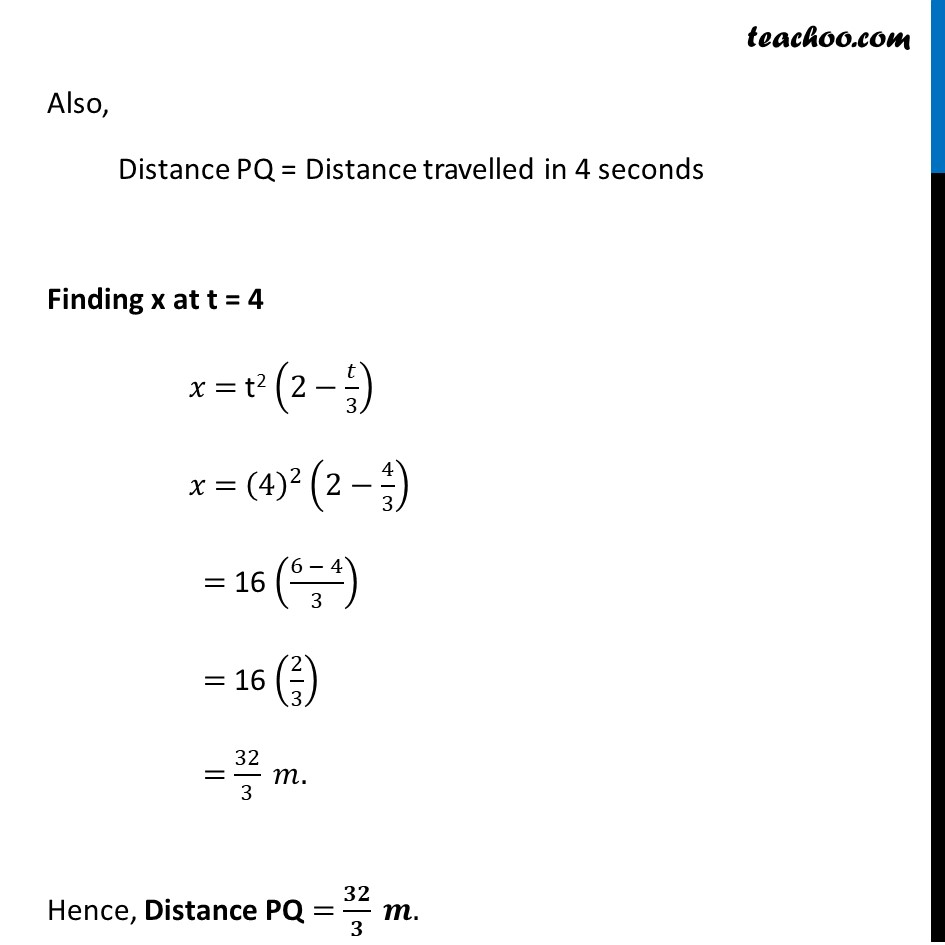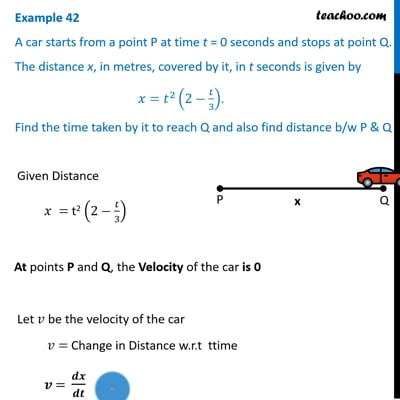Examples

Chapter 6 Class 12 Application of Derivatives
Serial order wiseThis video is only available for Teachoo black users

Maths Crash Course - Live lectures + all videos + Real time Doubt solving!

### Transcript

Example 42 A car starts from a point P at time t = 0 seconds and stops at point Q. The distance x, in metres, covered by it, in t seconds is given by 𝑥=𝑡^2 (2−𝑡/3). Find the time taken by it to reach Q and also find distance b/w P & Q Given Distance 𝑥 = t2 (2−𝑡/3) At points P and Q, the Velocity of the car is 0 Let 𝑣 be the velocity of the car 𝑣 = Change in Distance w.r.t ttime 𝒗 = 𝒅𝒙/𝒅𝒕 Finding 𝒗 𝑣 = 𝑑(𝑡^2 (2 − 𝑡/3))/𝑑𝑡 𝑣 = 𝑑(2𝑡^2− 𝑡^3/3)/𝑑𝑡 𝑣 = 4t – t2 Putting 𝒗 = 0 4t – t2 = 0 t(4−𝑡)=0 So, t = 0 & t = 4 Thus, it takes 4 seconds to reach from point P to Q Also, Distance PQ = Distance travelled in 4 seconds Finding x at t = 4 𝑥 = t2 (2−𝑡/3) 𝑥 = (4)^2 (2−4/3) = 16 ((6 − 4)/3) = 16 (2/3) = 32/3 𝑚. Hence, Distance PQ = 𝟑𝟐/𝟑 𝒎.